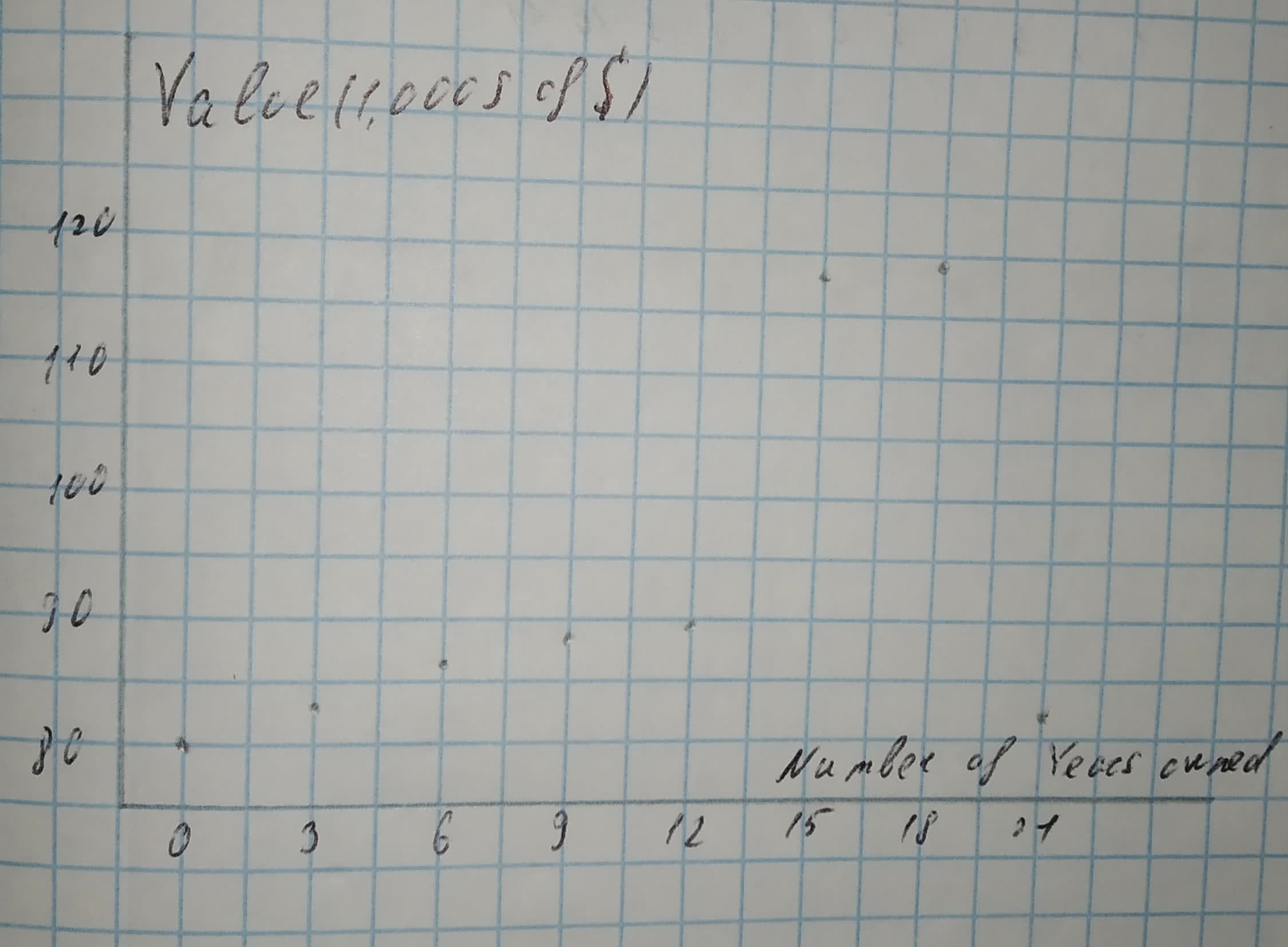Question# Make a scatterplot for the data in each table. Use the scatter plot to identify and clustering or outliers in the data. Value of Home Over Time Number

Normal distributions
ANSWEREDMake a scatterplot for the data in each table. Use the scatter plot to identify and clustering or outliers in the data.
Value of Home Over Time
Number of Years Owned: 0, 3, 6, 9, 12, 15, 18, 21
Value (1,000s of $): 80, 84, 86, 88, 89, 117, 119, 86 ## Answers (2)2021-05-20 Answer is given below (on video)2021-08-04 Step 1 Scatterplot Number of years owned is on the horizontal axis and Value (1,000s of$) is on the vertical axis.
The number of years owned range from 0 to 21, thus an appropriate scale for the horizontal axis is from —3 to 22.
The value (1,000s of \$) range from 80 to 119, thus an appropriate scale for the vertical axis is from 75 to 125.Step 2
There appear to be two outliers at 15 and 18 years, because the corresponding points lie far above the general pattern in the other points.
Result:
Two outliers.